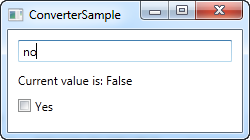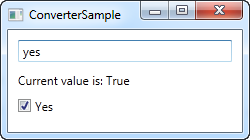Data binding:

# Value conversion with IValueConverter

So far we have used some simple data bindings, where the sending and receiving property was always compatible. However, you will soon run into situations where you want to use a bound value of one type and then present it slightly differently.

## When to use a value converter

Value converters are very frequently used with data bindings. Here are some basic examples:

• You have a numeric value but you want to show zero values in one way and positive numbers in another way
• You want to check a CheckBox based on a value, but the value is a string like "yes" or "no" instead of a Boolean value
• You have a file size in bytes but you wish to show it as bytes, kilobytes, megabytes or gigabytes based on how big it is

These are some of the simple cases, but there are many more. For instance, you may want to check a checkbox based on a Boolean value, but you want it reversed, so that the CheckBox is checked if the value is false and not checked if the value is true. You can even use a converter to generate an image for an ImageSource, based on the value, like a green sign for true or a red sign for false - the possibilities are pretty much endless!

For cases like this, you can use a value converter. These small classes, which implement the IValueConverter interface, will act like middlemen and translate a value between the source and the destination. So, in any situation where you need to transform a value before it reaches its destination or back to its source again, you likely need a converter.

## Implementing a simple value converter

As mentioned, a WPF value converter needs to implement the IValueConverter interface, or alternatively, the IMultiValueConverter interface (more about that one later). Both interfaces just requires you to implement two methods: Convert() and ConvertBack(). As the name implies, these methods will be used to convert the value to the destination format and then back again.

Let's implement a simple converter which takes a string as input and then returns a Boolean value, as well as the other way around. If you're new to WPF, and you likely are since you're reading this tutorial, then you might not know all of the concepts used in the example, but don't worry, they will all be explained after the code listings:

``````<Window x:Class="WpfTutorialSamples.DataBinding.ConverterSample"
xmlns="http://schemas.microsoft.com/winfx/2006/xaml/presentation"
xmlns:x="http://schemas.microsoft.com/winfx/2006/xaml"
xmlns:local="clr-namespace:WpfTutorialSamples.DataBinding"
Title="ConverterSample" Height="140" Width="250">
<Window.Resources>
<local:YesNoToBooleanConverter x:Key="YesNoToBooleanConverter" />
</Window.Resources>
<StackPanel Margin="10">
<TextBox Name="txtValue" />
<WrapPanel Margin="0,10">
<TextBlock Text="Current value is: " />
<TextBlock Text="{Binding ElementName=txtValue, Path=Text, Converter={StaticResource YesNoToBooleanConverter}}"></TextBlock>
</WrapPanel>
<CheckBox IsChecked="{Binding ElementName=txtValue, Path=Text, Converter={StaticResource YesNoToBooleanConverter}}" Content="Yes" />
</StackPanel>
</Window>``````
``````using System;
using System.Windows;
using System.Windows.Data;

namespace WpfTutorialSamples.DataBinding
{
public partial class ConverterSample : Window
{
public ConverterSample()
{
InitializeComponent();
}
}

public class YesNoToBooleanConverter : IValueConverter
{
public object Convert(object value, Type targetType, object parameter, System.Globalization.CultureInfo culture)
{
switch(value.ToString().ToLower())
{
case "yes":
case "oui":
return true;
case "no":
case "non":
return false;
}
return false;
}

public object ConvertBack(object value, Type targetType, object parameter, System.Globalization.CultureInfo culture)
{
if(value is bool)
{
if((bool)value == true)
return "yes";
else
return "no";
}
return "no";
}
}
}``````## Code-behind

So, let's start from the back and then work our way through the example. We have implemented a converter in the Code-behind file called YesNoToBooleanConverter. As advertised, it just implements the two required methods, called Convert() and ConvertBack(). The Convert() methods assumes that it receives a string as the input (the value parameter) and then converts it to a Boolean true or false value, with a fallback value of false. For fun, I added the possibility to do this conversion from French words as well.

The ConvertBack() method obviously does the opposite: It assumes an input value with a Boolean type and then returns the English word "yes" or "no" in return, with a fallback value of "no".

You may wonder about the additional parameters that these two methods take, but they're not needed in this example. We'll use them in one of the next chapters, where they will be explained.

## XAML

In the XAML part of the program, we start off by declaring an instance of our converter as a resource for the window. We then have a TextBox, a couple of TextBlocks and a CheckBox control and this is where the interesting things are happening: We bind the value of the TextBox to the TextBlock and the CheckBox control and using the Converter property and our own converter reference, we juggle the values back and forth between a string and a Boolean value, depending on what's needed.

If you try to run this example, you will be able to change the value in two places: By writing "yes" in the TextBox (or any other value, if you want false) or by checking the CheckBox. No matter what you do, the change will be reflected in the other control as well as in the TextBlock.

## Summary

This was an example of a simple value converter, made a bit longer than needed for illustrational purposes. In the next chapter we'll look into a more advanced example, but before you go out and write your own converter, you might want to check if WPF already includes one for the purpose. As of writing, there are more than 20 built-in converters that you may take advantage of, but you need to know their name. I found the following list which might come in handy for you: http://stackoverflow.com/questions/505397/built-in-wpf-ivalueconverters

This article has been fully translated into the following languages: